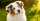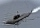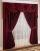# Calculator adding fractions and mixed numbers

The calculator performs basic and advanced operations with fractions, expressions with fractions combined with integers, decimals, and mixed numbers. It also shows detailed step-by-step information about the fraction calculation procedure. Solve problems with two, three, or more fractions and numbers in one expression.

### 8/5 + 62/7 = 276/35 = 7 31/35 ≅ 7.8857143

Spelled result in words is two hundred seventy-six thirty-fifths (or seven and thirty-one thirty-fifths).

### How do you solve fractions step by step?

1. Conversion a mixed number 6 2/7 to a improper fraction: 6 2/7 = 6 2/7 = 6 · 7 + 2/7 = 42 + 2/7 = 44/7

To find new numerator:
a) Multiply the whole number 6 by the denominator 7. Whole number 6 equally 6 * 7/7 = 42/7
b) Add the answer from previous step 42 to the numerator 2. New numerator is 42 + 2 = 44
c) Write a previous answer (new numerator 44) over the denominator 7.

Six and two sevenths is forty-four sevenths
2. Add: 8/5 + 44/7 = 8 · 7/5 · 7 + 44 · 5/7 · 5 = 56/35 + 220/35 = 56 + 220/35 = 276/35
For adding, subtracting, and comparing fractions, it is suitable to adjust both fractions to a common (equal, identical) denominator. The common denominator you can calculate as the least common multiple of both denominators - LCM(5, 7) = 35. In practice, it is enough to find the common denominator (not necessarily the lowest) by multiplying the denominators: 5 × 7 = 35. In the next intermediate step, the fraction result cannot be further simplified by canceling.
In words - eight fifths plus forty-four sevenths = two hundred seventy-six thirty-fifths.

#### Rules for expressions with fractions:

Fractions - use the slash “/” between the numerator and denominator, i.e., for five-hundredths, enter 5/100. If you are using mixed numbers, be sure to leave a single space between the whole and fraction part.
The slash separates the numerator (number above a fraction line) and denominator (number below).

Mixed numerals (mixed fractions or mixed numbers) write as non-zero integer separated by one space and fraction i.e., 1 2/3 (having the same sign). An example of a negative mixed fraction: -5 1/2.
Because slash is both signs for fraction line and division, we recommended use colon (:) as the operator of division fractions i.e., 1/2 : 3.

Decimals (decimal numbers) enter with a decimal point . and they are automatically converted to fractions - i.e. 1.45.

The colon : and slash / is the symbol of division. Can be used to divide mixed numbers 1 2/3 : 4 3/8 or can be used for write complex fractions i.e. 1/2 : 1/3.
An asterisk * or × is the symbol for multiplication.
Plus + is addition, minus sign - is subtraction and ()[] is mathematical parentheses.
The exponentiation/power symbol is ^ - for example: (7/8-4/5)^2 = (7/8-4/5)2

The calculator follows well-known rules for order of operations. The most common mnemonics for remembering this order of operations are:
PEMDAS - Parentheses, Exponents, Multiplication, Division, Addition, Subtraction.
BEDMAS - Brackets, Exponents, Division, Multiplication, Addition, Subtraction
BODMAS - Brackets, Of or Order, Division, Multiplication, Addition, Subtraction.
GEMDAS - Grouping Symbols - brackets (){}, Exponents, Multiplication, Division, Addition, Subtraction.
Be careful, always do multiplication and division before addition and subtraction. Some operators (+ and -) and (* and /) has the same priority and then must evaluate from left to right.

## Fractions in word problems:

• ExpressionsLet k represent an unknown number, express the following expressions: 1. The sum of the number n and two 2. The quotient of the numbers n and nine 3. Twice the number n 4. The difference between nine and the number n 5. Nine less than the number n
• Simplify 3Simplify mixed numerals expression: 8 1/4- 3 2/5 - (2 1/3 - 1/4) Show your solution.
• An orchardDuring a visit to an orchard, Greg picked 3/5 of a bag of delicious golden apples, 4/5 of a bag of Macintosh apples, 2/5 of a bag of Cortland apples, 1/5 of a bag of Bartlett pears, and 4/5 of a bag of Bosch pears. How many bags of fruit to Greg pick in t
• Circular gardenAlice creates a circular vegetable garden. Tomatoes are planted in 1/3 of the circular garden, carrots are planted in 2/5 of the circular garden, and green peppers are planted in 1/10 of the circular garden. What fraction represents the remaining unplante
• Weigh in totalI put 3/5 kg of grapes into a box which is 1/4kg in weight. How many kilograms do the grapes and the box weigh in total?
• Math testBrayden was solving some math problems for the math team. He answered 2 math problems. Matthew answered 3, John answered 1 reasoning. Matthew 1/2 times as many. Brayden said that 2/6. Is he correct? Why or why not? Be sure to explain your answer.
• BaxterBaxter ate 1/12 of a box of dog food. Now the box is 3/4 full. What fraction of the full of a full box was there before Baxter?
• Length subtractingExpress in mm: 5 3/10 cm - 2/5 mm
• Evaluate 17Evaluate 2x+6y when x=- 4/5 and y=1/3. Write your answer as a fraction or mixed number in simplest form.
• SubmergingMonika dove 9 meters below the ocean's surface. She then dove 13 meters deeper. Then she rose 19 and one-fourth meters. What was her position concerning the water's surface (the water surface = 0, minus values = above water level, plus = above water level
• Interior designerTo make draperies an interior designer needs 11 1/4 yards of material for the den and 8 1/2 yards for the living room. If material comes only in 20 yard bolts, how much will be left over after completing both sets of draperies?
• Flowers 4A flower seller has 4.05 kg of orange flowers and 6.50 kg of red flowers. He made flower baskets with 2.11 kg of both red and orange flowers in each. How many baskets did he make?
• Sum of fractionsWhat is the sum of 2/3+3/5?